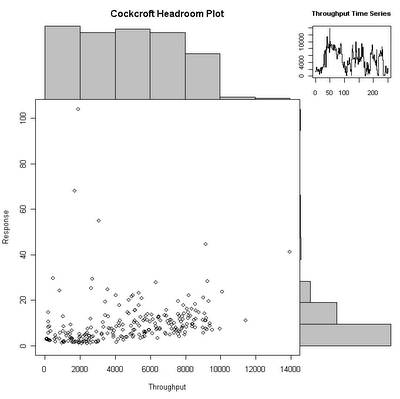## Monday, November 20, 2006

### Cockcroft Headroom Plot - Part 2 - R Version

I kept tweaking the code, and came up with a prettier version, that also has a small time series view of the throughput in the top right corner.The code for this is
`chp <- function(x,y,xl="Throughput",yl="Response",tl="Throughput Time Series", ml="Cockcroft Headroom Plot") {       xhist <- hist(x,plot=FALSE)       yhist <- hist(y, plot=FALSE)       xrange <- c(0,max(x))       yrange <- c(0,max(y))       nf <- layout(matrix(c(2,4,1,3),2,2,byrow=TRUE), c(3,1), c(1,3), TRUE)       layout.show(nf)       par(mar=c(5,4,0,0))       plot(x, y, xlim=xrange, ylim=yrange, xlab=xl, ylab=yl)       par(mar=c(0,4,3,0))       barplot(xhist\$counts, axes=FALSE, ylim=c(0, max(xhist\$counts)), space=0, main=ml)       par(mar=c(5,0,0,1))       barplot(yhist\$counts, axes=FALSE, xlim=c(0,max(yhist\$counts)), space=0, horiz=TRUE)       par(mar=c(2.5,1.5,3,1))       plot(x, main=tl, cex.axis=0.8, cex.main=0.8, type="S")}`

I also made a wrapper function that steps through the data over time in chunks.
`> chp.step <- function(x, y, steps=10, secs=1.0) {    xl <- length(x)    step <- xl/steps    for(n in 0:(steps-1)) {        Sys.sleep(secs)        chp(x[(1+n*step):min((n+1)*step,xl)],y[(1+n*step):min((n+1)*step,xl)])    }}`

To run this smoothly on windows, I had to disable double buffering using
`> options("windowsBuffered"=FALSE)`

and close the graphics window so that a new one opens with the new option.

The data is displayed using the same calls as described in Part 1. The next step is to try some different data sets and work on detecting saturation automatically.

#### 2 comments:

1.I really like the headroom plot.

I'm just curious if you can figure out how to get the time series of response displayed as well.

2.If you substitute "y" instead of "x" for the last call to plot it will show response time. The default title should also be changed. Its also easy to code this as a parameter. However I can't see any space on the plot to fit both resp and throughput vs. time, it would mean quite a lot of rearrangement, and its very easy to get a plot vs. time using plot(r.s+w.s) or plot(asvc_t+wsvc_t) at the prompt.

Note: Only a member of this blog may post a comment.